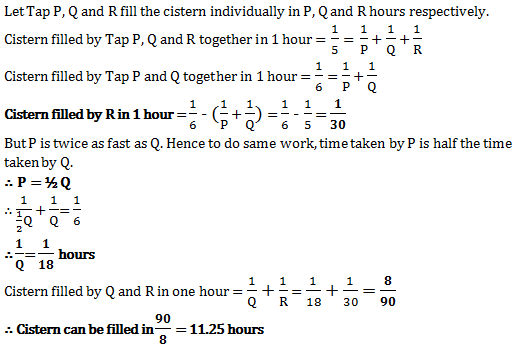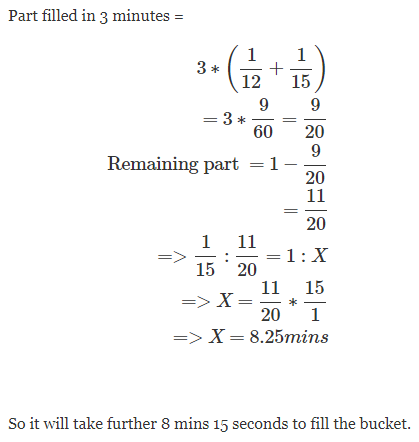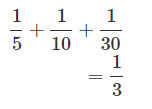Top

# Pipes & Cistern

1.

12 buckets of water fill a tank when the capacity of each tank is 13.5 litres. How many buckets will be needed to fill the same tank, if the capacity of each bucket is 9 litres?

Capacity of the tank = (12*13.5) litres

= 162 litres

Capacity of each bucket = 9 litres.

So we can get answer by dividing total capacity of tank by total capacity of bucket.

Number of buckets needed = (162/9) = 18 buckets

Enter details here

2.

Three pipes A, B and C are connected to a tank. Working alone, they require 10 hours, 20 hours and 30 hours respectively. After some time, A is closed and after another 2 hours, B is also closed. C works for another 14 hours so that the tank gets filled completely. Find the time (in hours) after which pipe A was closed.

Let the capacity of the tank be LCM (10, 20, 30) = 60
=> Efficiency of pipe A = 60 / 10 = 6 units / hour
=> Efficiency of pipe B = 60 / 20 = 3 units / hour
=> Efficiency of pipe C = 60 / 30 = 2 units / hour
Now, all three work for some time, say ‘t’ hours.
So, B and C work for 2 more hours after ‘t’ hours and then, C works for another 14 hours.
=> Combined efficiency of pipe A, pipe B and pipe C = 11 units / hour
=> Combined efficiency of pipe B and pipe C = 5 units / hour

So, we have 11 x t + 5 x 2 + 14 x 2 = 60
=> 11 t + 10 + 28 = 60
=> 11 t = 60 – 38
=> 11 t = 22
=> t = 2

Therefore, A was closed after 2 hours.

Enter details here

3.

A pump can fill a tank with water in 2 hours. Because of a leak, it took 2 hours to fill the tank. The leak can drain all the water of the tank in:

Work done by the leak in 1 hour = (1/2 - 3/7) = 1/4

Leak will empty the tank in 14 hrs.

Enter details here

4.

If a pipe can fill a tank in 6 hours, find the part of tank it fills in one hour.

Let the capacity of the tank be C litres.
Time to fill the tank = 6 hours
Hence, in 1 hour C/6 litres gets filled.
Therefore, 1/6th part of the tank gets filled in one hour.

Enter details here

5.

Pipes A and B fill a tank in 5 and 6 hours respectively. Pipe C empties the tank in 12 hours, if you open all the three pipes together this tank will be filled in?

It is given that Pipe A,B and C work simultaneously.

Pipe A and B are filling the tank and Pipe C is emptying it.

In 1 hour volume of tank filled by Pipe A: 1/5th

In 1 hour volume of tank filled by Pipe B: 1/6th

In 1 hour volume of tank emptied by Pipe C: 1/12th

Working together Pipe A B and C fill x volume of tank.

=>1/x = (1/5)+(1/6)-(1/12)

=>x=60/17 hours

Enter details here

6.

A cistern can be filled in 6 hours by taps P and Q. If tap R also joins them, then cistern is filled in 5 hours. Tap P can fill the cistern at twice the rate of tap Q. In what time tap Q and R fill the cistern?Enter details here

7.

Taps A and B can fill a bucket in 12 minutes and 15 minutes respectively. If both are opened and A is closed after 3 minutes, how much further time would it take for B to fill the bucket?Enter details here

8.

A pump can fill a tank with water in 2 hours. Because of a leak, it took 2 1/3 hours to fill the tank. The leak can drain all the water of the tank in .. ?

No answer description available for this question.

Enter details here

9.

Pipe A can fill a tank in 5 hours, pipe B in 10 hours and pipe C in 30 hours. If all the pipes are open, in how many hours will the tank be filled ?

Part filled by A in 1 hour = 1/5

Part filled by B in 1 hour = 1/10

Part filled by C in 1 hour = 1/30

Part filled by (A+B+C) in 1 hour =So all pipes will fill the tank in 3 hours.

Enter details here

10.

In 6 hours a tank is filled. After the tank is half filled, 3 more similar taps are opened. Estimate the total time the tank will take to fill.